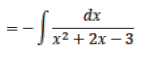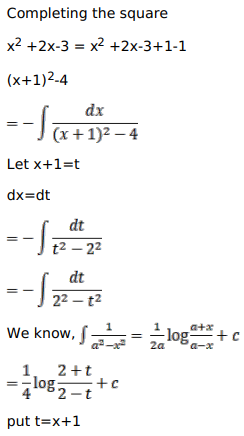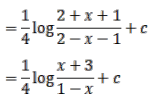# Solve this followingQuestion:

Mark $(\sqrt{)}$ against the correct answer in each of the following:

$\int \frac{\mathrm{dx}}{\left(3-2 \mathrm{x}-\mathrm{x}^{2}\right)}=?$

A. $\frac{1}{4} \log \left|\frac{3+x}{3-x}\right|+C$

B. $\frac{1}{4} \log \left|\frac{1+x}{1-x}\right|+C$

C. $\frac{1}{4} \log \left|\frac{3+x}{1-x}\right|+C$

D. none of these

Solution: